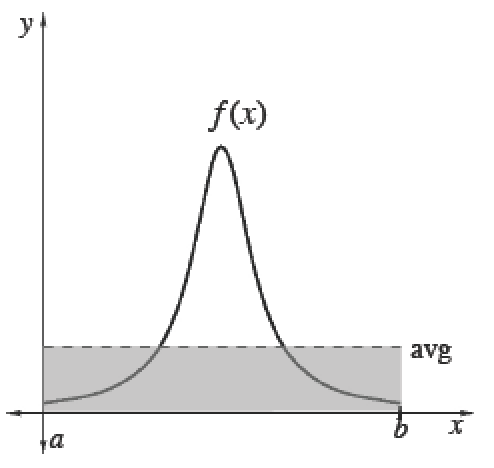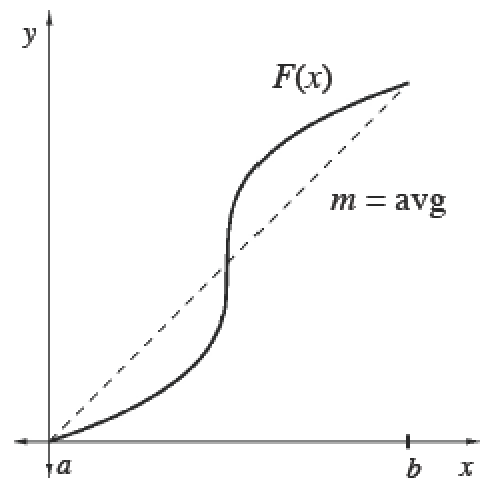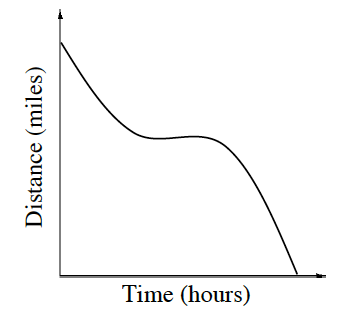### Home > APCALC > Chapter 7 > Lesson 7.3.2 > Problem7-116

7-116.

What if the graph at right represents the velocity in miles per hour of the shuttle? In this case, how would you determine the average velocity? Describe the method you would use.

Given a velocity function, will average velocity be represented by 'average area under the curve' or 'average rate of change'?

Average (Mean) Values

 To calculate the mean (average) value of a finite set of items, add up the values of items and divide by the number of items.Integrals help us add over a continuous interval. Therefore, for any continuous function $f$ :$\frac{\int_a^bf(x)dx}{b-a}=$ mean value of $f$ over $[a, b]$Since $\int _ { a } ^ { b } f ( x ) d x$, we can also calculate the average value of any function $f$ using its antiderivative $F$. Its average slope gives the average rate of change of $F$, which is the same as the average value of $f.$$\frac{\int_a^bf(x)dx}{b-a}=\frac { F ( b ) - F ( a ) } { b - a }=$  mean rate of change of $F ′$ over $[a, b]$